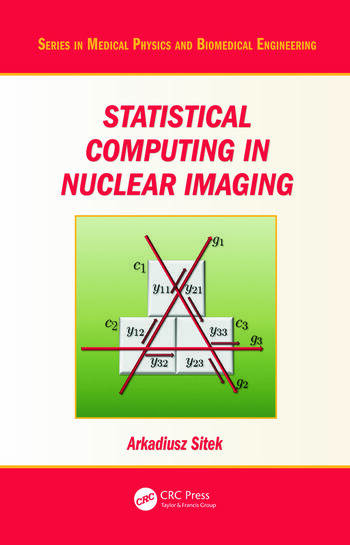# Statistical Computing in Nuclear Imaging

## 1st Edition

CRC Press
Published December 17, 2014
Reference - 275 Pages - 76 B/W Illustrations
ISBN 9781439849347 - CAT# K12233
Series: Series in Medical Physics and Biomedical Engineering

For Instructors Request Inspection Copy

USD\$145.00

FREE Standard Shipping!

## Preview

### Summary

Statistical Computing in Nuclear Imaging introduces aspects of Bayesian computing in nuclear imaging. The book provides an introduction to Bayesian statistics and concepts and is highly focused on the computational aspects of Bayesian data analysis of photon-limited data acquired in tomographic measurements.

Basic statistical concepts, elements of decision theory, and counting statistics, including models of photon-limited data and Poisson approximations, are discussed in the first chapters. Monte Carlo methods and Markov chains in posterior analysis are discussed next along with an introduction to nuclear imaging and applications such as PET and SPECT.

The final chapter includes illustrative examples of statistical computing, based on Poisson-multinomial statistics. Examples include calculation of Bayes factors and risks as well as Bayesian decision making and hypothesis testing. Appendices cover probability distributions, elements of set theory, multinomial distribution of single-voxel imaging, and derivations of sampling distribution ratios. C++ code used in the final chapter is also provided.

The text can be used as a textbook that provides an introduction to Bayesian statistics and advanced computing in medical imaging for physicists, mathematicians, engineers, and computer scientists. It is also a valuable resource for a wide spectrum of practitioners of nuclear imaging data analysis, including seasoned scientists and researchers who have not been exposed to Bayesian paradigms.

#### Instructors

We provide complimentary e-inspection copies of primary textbooks to instructors considering our books for course adoption.